# 7  Introduction to sf and stars

This chapter introduces R packages sf and stars. sf provides a table format for simple features, where feature geometries are stored in a list-column. R package stars was written to support raster and vector data cubes (Chapter 6), supporting raster layers, raster stacks and feature time series as special cases. sf first appeared on CRAN in 2016, stars in 2018. Development of both packages received support from the R Consortium as well as strong community engagement. The packages were designed to work together. Functions or methods operating on sf or stars objects start with st_, making it easy to recognise them or to search for them when using command line completion.

## 7.1 Package sf

Intended to succeed and replace R packages sp, rgeos and the vector parts of rgdal, R package sf was developed to move spatial data analysis in R closer to standards-based approaches seen in the industry and open source projects, to build upon more modern versions of the open source geospatial software stack (Figure 1.7), and to allow for integration of R spatial software with the tidyverse , if desired.

To do so, R package sf provides simple features access , natively, to R. It provides an interface to several tidyverse packages, in particular to ggplot2, dplyr, and tidyr. It can read and write data through GDAL, execute geometrical operations using GEOS (for projected coordinates) or s2geometry (for ellipsoidal coordinates), and carry out coordinate transformations or conversions using PROJ. External C++ libraries are interfaced using R package Rcpp .

Package sf represents sets of simple features in sf objects, a sub-class of a data.frame or tibble. sf objects contain at least one geometry list-column of class sfc, which for each element contains the geometry as an R object of class sfg. A geometry list-column acts as a variable in a data.frame or tibble, but has a more complex structure than basic vectors such as numeric or character variables Section B.3.

An sf object has the following metadata:

• the name of the (active) geometry column, held in attribute sf_column
• for each non-geometry variable, the attribute-geometry relationship (Section 5.1), held in attribute agr

An sfc geometry list-column is extracted from an sf object with st_geometry and has the following metadata:

• coordinate reference system held in attribute crs
• bounding box held in attribute bbox
• precision held in attribute precision
• number of empty geometries held in attribute n_empty

These attributes may best be accessed or set by using functions like st_bbox, st_crs, st_set_crs, st_agr, st_set_agr, st_precision, and st_set_precision.

Geometry columns in sf objects can be set or replaced using st_geometry<- or st_set_geometry.

### Creation

An sf object can be created from scratch by

library(sf)
# Linking to GEOS 3.11.1, GDAL 3.6.4, PROJ 9.1.1; sf_use_s2() is TRUE
p1 <- st_point(c(7.35, 52.42))
p2 <- st_point(c(7.22, 52.18))
p3 <- st_point(c(7.44, 52.19))
sfc <- st_sfc(list(p1, p2, p3), crs = 'OGC:CRS84')
st_sf(elev = c(33.2, 52.1, 81.2),
marker = c("Id01", "Id02", "Id03"), geom = sfc)
# Simple feature collection with 3 features and 2 fields
# Geometry type: POINT
# Dimension:     XY
# Bounding box:  xmin: 7.22 ymin: 52.2 xmax: 7.44 ymax: 52.4
# Geodetic CRS:  WGS 84
#   elev marker              geom
# 1 33.2   Id01 POINT (7.35 52.4)
# 2 52.1   Id02 POINT (7.22 52.2)
# 3 81.2   Id03 POINT (7.44 52.2)

Figure 7.1 gives an explanation of the components printed. Rather than creating objects from scratch, spatial data in R are typically read from an external source, which can be:

• external file
• table (or set of tables) in a database
• request to a web service
• dataset held in some form in another R package

The next section introduces reading from files. Section 9.1 discusses handling of datasets too large to fit into working memory.

Reading datasets from an external “data source” (file, web service, or even string) is done using st_read:

library(sf)
(file <- system.file("gpkg/nc.gpkg", package = "sf"))
#  "/home/edzer/R/x86_64-pc-linux-gnu-library/4.3/sf/gpkg/nc.gpkg"
# Reading layer nc.gpkg' from data source
#   /home/edzer/R/x86_64-pc-linux-gnu-library/4.3/sf/gpkg/nc.gpkg'
#   using driver GPKG'
# Simple feature collection with 100 features and 14 fields
# Geometry type: MULTIPOLYGON
# Dimension:     XY
# Bounding box:  xmin: -84.3 ymin: 33.9 xmax: -75.5 ymax: 36.6
# Geodetic CRS:  NAD27

Here, the file name and path file is read from the sf package, which has a different path on every machine, and hence is guaranteed to be present on every sf installation.

Command st_read has two arguments: the data source name (dsn) and the layer. In the example above, the geopackage (GPKG) file contains only a single layer that is being read. If it had contained multiple layers, then the first layer would have been read and a warning would have been emitted. The available layers of a dataset can be queried by

st_layers(file)
# Driver: GPKG
# Available layers:
#   layer_name geometry_type features fields crs_name
# 1    nc.gpkg Multi Polygon      100     14    NAD27

Simple feature objects can be written with st_write, as in

(file = tempfile(fileext = ".gpkg"))
#  "/tmp/Rtmpm9lGRF/file361e653fae4a9.gpkg"
st_write(nc, file, layer = "layer_nc")
# Writing layer layer_nc' to data source
#   /tmp/Rtmpm9lGRF/file361e653fae4a9.gpkg' using driver GPKG'
# Writing 100 features with 14 fields and geometry type Multi Polygon.

where the file format (GPKG) is derived from the file name extension. Using argument append, st_write can either append records to an existing layer or replace it; if unset, it will err if a layer already exists. The tidyverse-style write_sf will replace silently if append has not been set. Layers can also be deleted using st_delete, which is convenient in particular when they are associated with tables in a database.

For file formats supporting a WKT-2 coordinate reference system, sf_read and sf_write will read and write it. For simple formats such as csv this will not work. The shapefile format supports only a very limited encoding of the CRS.

### Subsetting

A very common operation is to subset objects; base R can use [ for this. The rules that apply to data.frame objects also apply to sf objects: records 2-5 and columns 3-7 are selected by

nc[2:5, 3:7]

but with a few additional features, in particular:

• the drop argument is by default FALSE meaning that the geometry column is always selected, and an sf object is returned; when it is set to TRUE and the geometry column is not selected, it is dropped and a data.frame is returned
• selection with a spatial (sf, sfc or sfg) object as first argument leads to selection of the features that spatially intersect with that object (see next section); other predicates than intersects can be chosen by setting parameter op to a function such as st_covers or any other binary predicate function listed in Section 3.2.2.

### Binary predicates

Binary predicates like st_intersects, st_covers and such (Section 3.2.2) take two sets of features or feature geometries and return for all pairs whether the predicate is TRUE or FALSE. For large sets this would potentially result in a huge matrix, typically filled mostly with FALSE values and for that reason a sparse representation is returned by default:

nc5 <- nc[1:5, ]
nc7 <- nc[1:7, ]
(i <- st_intersects(nc5, nc7))
# Sparse geometry binary predicate list of length 5, where the
# predicate was intersects'
#  1: 1, 2
#  2: 1, 2, 3
#  3: 2, 3
#  4: 4, 7
#  5: 5, 6
Code
plot(st_geometry(nc7))
plot(st_geometry(nc5), add = TRUE, border = "brown")
cc = st_coordinates(st_centroid(st_geometry(nc7)))
text(cc, labels = 1:nrow(nc7), col = "blue")

Figure 7.2 shows how the intersections of the first five with the first seven counties can be understood. We can transform the sparse logical matrix into a dense matrix by

as.matrix(i)
#       [,1]  [,2]  [,3]  [,4]  [,5]  [,6]  [,7]
# [1,]  TRUE  TRUE FALSE FALSE FALSE FALSE FALSE
# [2,]  TRUE  TRUE  TRUE FALSE FALSE FALSE FALSE
# [3,] FALSE  TRUE  TRUE FALSE FALSE FALSE FALSE
# [4,] FALSE FALSE FALSE  TRUE FALSE FALSE  TRUE
# [5,] FALSE FALSE FALSE FALSE  TRUE  TRUE FALSE

The number of counties that each of nc5 intersects with is

lengths(i)
#  2 3 2 2 2

and the other way around, the number of counties in nc5 that intersect with each of the counties in nc7 is

lengths(t(i))
#  2 3 2 1 1 1 1

The object i is of class sgbp (sparse geometrical binary predicate), and is a list of integer vectors, with each element representing a row in the logical predicate matrix holding the column indices of the TRUE values for that row. It further holds some metadata like the predicate used, and the total number of columns. Methods available for sgbp objects include

methods(class = "sgbp")
#   as.data.frame as.matrix     coerce        dim
#   initialize    Ops           print         show
#   slotsFromS3   t
# see '?methods' for accessing help and source code

where the only Ops method available is !, the negation operation.

### tidyverse

The tidyverse package loads a collection of data science packages that work well together . Package sf has tidyverse-style read and write functions, read_sf and write_sf that

• return a tibble rather than a data.frame,
• do not print any output, and
• overwrite existing data by default.

Further tidyverse generics with methods for sf objects include filter, select, group_by, ungroup, mutate, transmute, rowwise, rename, slice, summarise, distinct, gather, pivot_longer, spread, nest, unnest, unite, separate, separate_rows, sample_n, and sample_frac. Most of these methods simply manage the metadata of sf objects and make sure the geometry remains present. In case a user wants the geometry to be removed, one can use st_drop_geometry or simply coerce to a tibble or data.frame before selecting:

library(tidyverse) |> suppressPackageStartupMessages()
nc |> as_tibble() |> select(BIR74) |> head(3)
# # A tibble: 3 × 1
#   BIR74
#   <dbl>
# 1  1091
# 2   487
# 3  3188

The summarise method for sf objects has two special arguments:

• do_union (default TRUE) determines whether grouped geometries are unioned on return, so that they form a valid geometry
• is_coverage (default FALSE) in case the geometries grouped form a coverage (do not have overlaps), setting this to TRUE speeds up the unioning

The distinct method selects distinct records, where st_equals is used to evaluate distinctness of geometries.

filter can be used with the usual predicates; when one wants to use it with a spatial predicate, for instance to select all counties less than 50 km away from Orange County, one could use

orange <- nc |> dplyr::filter(NAME == "Orange")
wd <- st_is_within_distance(nc, orange,
units::set_units(50, km))
o50 <- nc |> dplyr::filter(lengths(wd) > 0)
nrow(o50)
#  17

(where we use dplyr::filter rather than filter to avoid confusion with filter from base R.)

Figure 7.3 shows the results of this analysis, and in addition a buffer around the county borders. Note that this buffer serves for illustration: it was not used to select the counties.

Code
og <- st_geometry(orange)
buf50 <- st_buffer(og, units::set_units(50, km))
all <- c(buf50, st_geometry(o50))
plot(st_geometry(o50), lwd = 2, extent = all)
plot(og, col = 'orange', add = TRUE)
plot(buf50, add = TRUE, col = NA, border = 'brown')
plot(st_geometry(nc), add = TRUE, border = 'grey')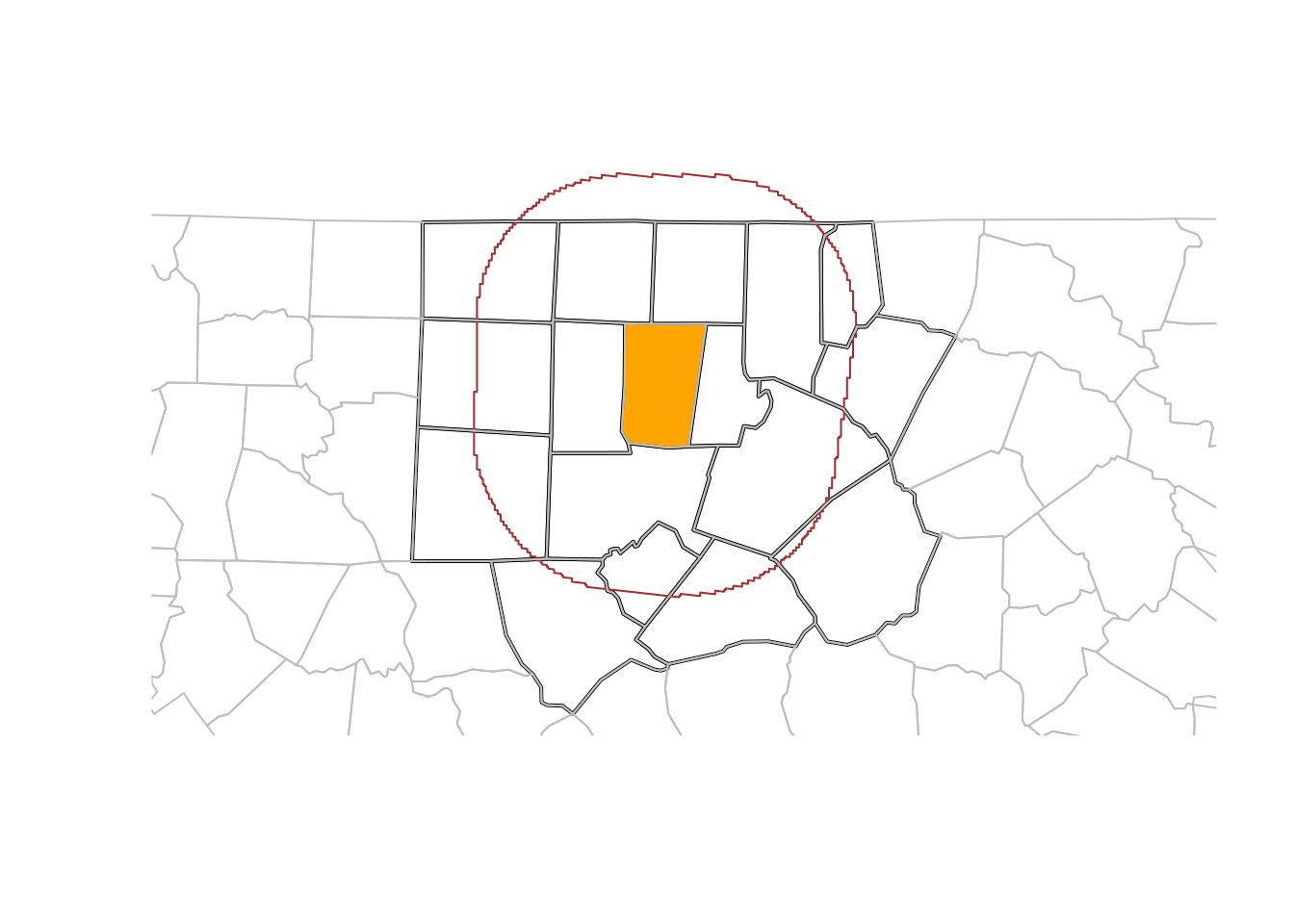Figure 7.3: Orange County (orange), counties within a 50 km radius (black), a 50~km buffer around Orange County (brown), and remaining counties (grey)

## 7.2 Spatial joins

In regular (left, right, or inner) joins, joined records from a pair of tables are reported when one or more selected attributes match (are identical) in both tables. A spatial join is similar, but the criterion to join records is not equality of attributes but a spatial predicate. This leaves a wide variety of options in order to define spatially matching records, using binary predicates listed in Section 3.2.2. The concepts of “left”, “right”, “inner”, or “full” joins remain identical to the non-spatial join as the options for handling records that have no spatial match.

When using spatial joins, each record may have several matched records, yielding a large result table. A way to reduce this complexity may be to select from the matching records the one with the largest overlap with the target geometry. An example of this is shown (visually) in Figure 7.4; this is done using st_join with argument largest = TRUE.

Code
# example of largest = TRUE:
system.file("shape/nc.shp", package="sf") |>
st_transform('EPSG:2264') -> nc
gr <- st_sf(
label = apply(expand.grid(1:10, LETTERS[10:1])[,2:1], 1, paste0, collapse = ""),
geom = st_make_grid(nc))
gr$col <- sf.colors(10, categorical = TRUE, alpha = .3) # cut, to verify that NA's work out: gr <- gr[-(1:30),] suppressWarnings(nc_j <- st_join(nc, gr, largest = TRUE)) par(mfrow = c(2,1), mar = rep(0,4)) plot(st_geometry(nc_j), border = 'grey') plot(st_geometry(gr), add = TRUE, col = gr$col)
text(st_coordinates(st_centroid(st_geometry(gr))), labels = gr$label, cex = .85) # the joined dataset: plot(st_geometry(nc_j), border = 'grey', col = nc_j$col)
trncls[c(8, 14, 15, 18, 19)] <- "water" model <- MASS::lda(cls ~ ., st_drop_geometry(trn)) pr <- predict(rs, model) Here, we used the MASS:: prefix to avoid loading MASS, as that would mask select from dplyr. The split step is needed to convert the band dimension into attributes, so that they are offered as a set of predictors. Code plot(pr, key.pos = 4, key.width = lcm(3.5), key.length = lcm(2))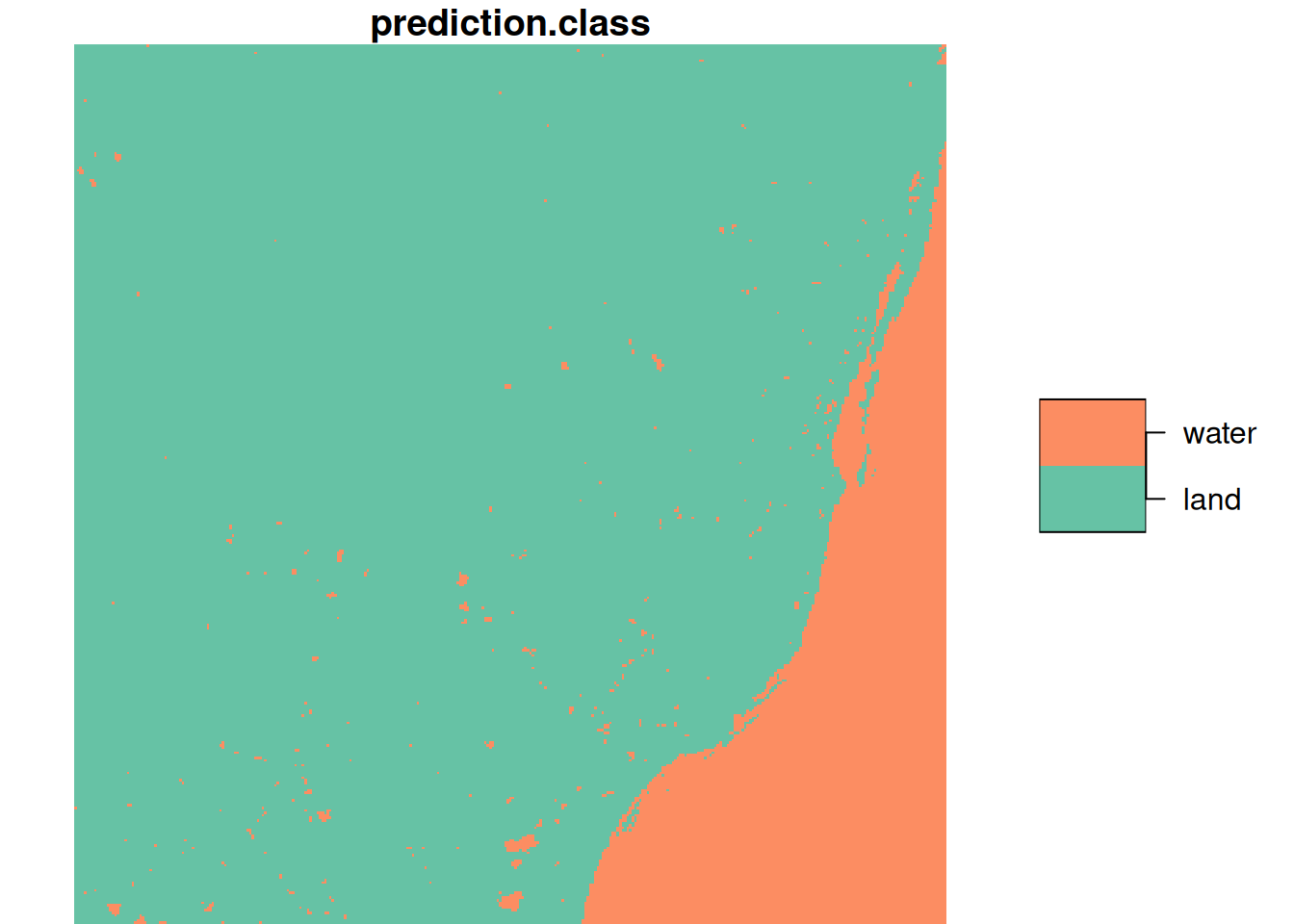Figure 7.8: Linear discriminant classifier for land/water, based on training data of Figure 7.7 We also see that the layer plotted in Figure 7.8 is a factor variable, with class labels. ### Plotting raster data Code plot(r)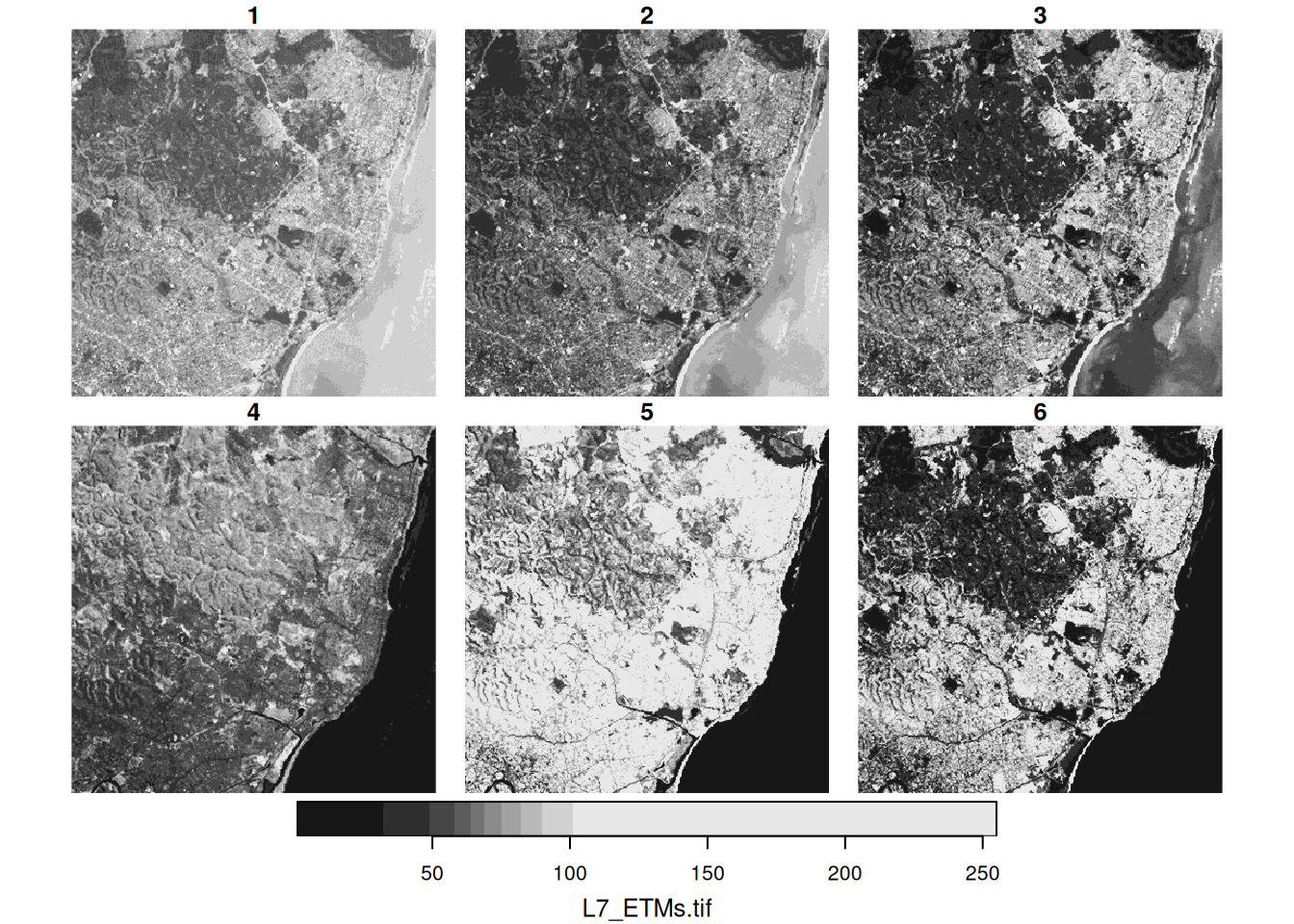Figure 7.9: Six 30 m Landsat bands downsampled to 90m for Olinda, Br. We can use the base plot method for stars objects, where the plot created with plot(r) is shown in Figure 7.9. The default colour scale uses grey tones and stretches them such that colour breaks correspond to data quantiles over all bands (“histogram equalization”). Setting breaks = "equal" gives equal width colour breaks; alternatively a sequence of numeric break values can be given. A more familiar view may be the RGB or false colour composite shown in Figure 7.10. Code par(mfrow = c(1, 2)) plot(r, rgb = c(3,2,1), reset = FALSE, main = "RGB") # rgb plot(r, rgb = c(4,3,2), main = "False colour (NIR-R-G)") # false colour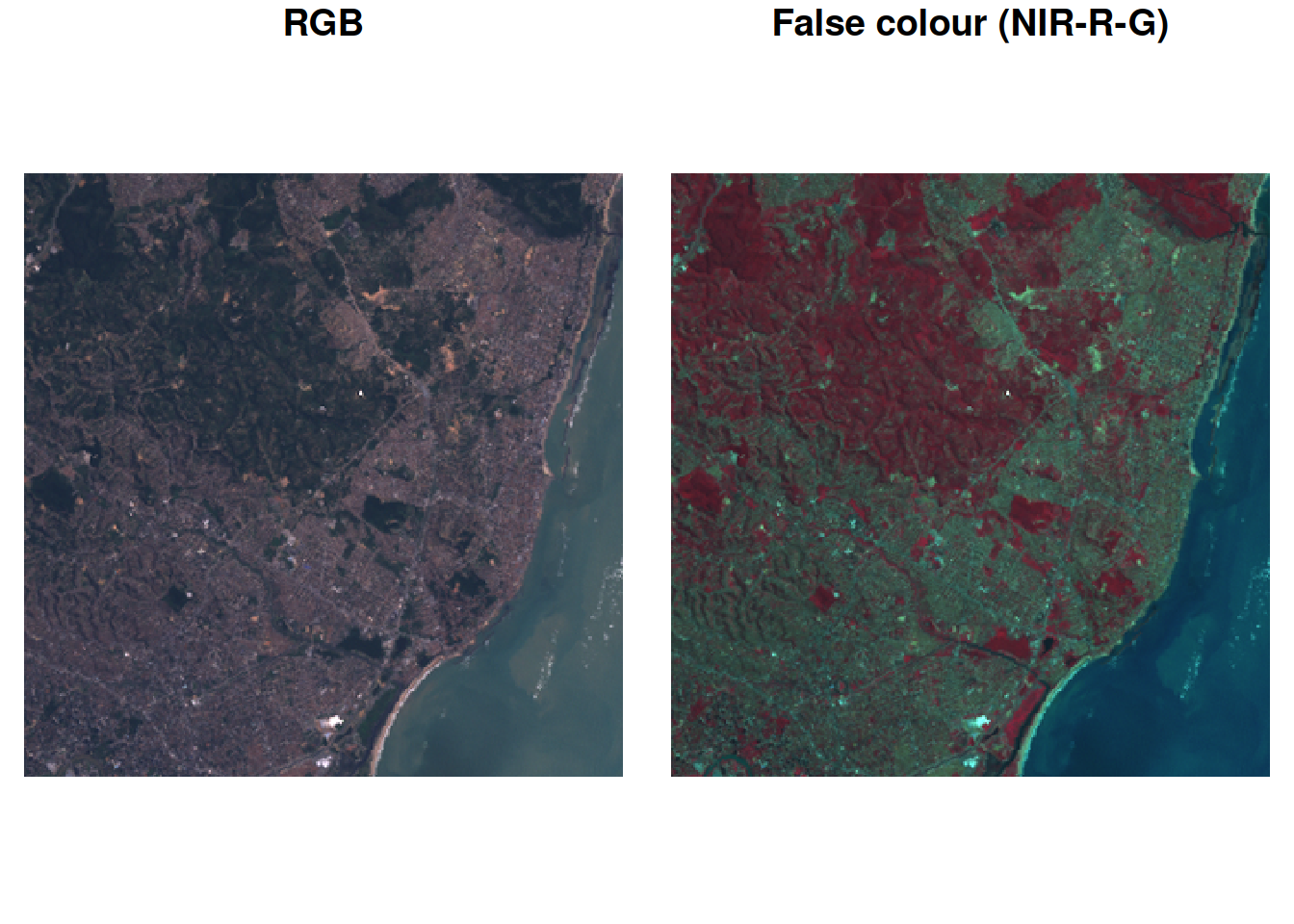Figure 7.10: Two colour composites Further details and options are given in Chapter 8. ### Analysing raster data Element-wise mathematical functions (Section 6.3.2) on stars objects are just passed on to the arrays. This means that we can call functions and create expressions: log(r) # stars object with 3 dimensions and 1 attribute # attribute(s): # Min. 1st Qu. Median Mean 3rd Qu. Max. # L7_ETMs.tif 0 3.99 4.23 4.12 4.45 5.54 # dimension(s): # from to offset delta refsys point x/y # x 1 349 288776 28.5 SIRGAS 2000 / ... FALSE [x] # y 1 352 9120761 -28.5 SIRGAS 2000 / ... FALSE [y] # band 1 6 NA NA NA NA r + 2 * log(r) # stars object with 3 dimensions and 1 attribute # attribute(s): # Min. 1st Qu. Median Mean 3rd Qu. Max. # L7_ETMs.tif 1 62 77.5 77.1 94.9 266 # dimension(s): # from to offset delta refsys point x/y # x 1 349 288776 28.5 SIRGAS 2000 / ... FALSE [x] # y 1 352 9120761 -28.5 SIRGAS 2000 / ... FALSE [y] # band 1 6 NA NA NA NA or even mask out certain values: r2 <- r r2[r < 50] <- NA r2 # stars object with 3 dimensions and 1 attribute # attribute(s): # Min. 1st Qu. Median Mean 3rd Qu. Max. NA's # L7_ETMs.tif 50 64 75 79 90 255 149170 # dimension(s): # from to offset delta refsys point x/y # x 1 349 288776 28.5 SIRGAS 2000 / ... FALSE [x] # y 1 352 9120761 -28.5 SIRGAS 2000 / ... FALSE [y] # band 1 6 NA NA NA NA or unmask areas: r2[is.na(r2)] <- 0 r2 # stars object with 3 dimensions and 1 attribute # attribute(s): # Min. 1st Qu. Median Mean 3rd Qu. Max. # L7_ETMs.tif 0 54 69 63 86 255 # dimension(s): # from to offset delta refsys point x/y # x 1 349 288776 28.5 SIRGAS 2000 / ... FALSE [x] # y 1 352 9120761 -28.5 SIRGAS 2000 / ... FALSE [y] # band 1 6 NA NA NA NA Dimension-wise, we can apply functions to selected array dimensions (Section 6.3.3) of stars objects similar to how apply does this to arrays. For instance, we can compute for each pixel the mean of the six band values by st_apply(r, c("x", "y"), mean) # stars object with 2 dimensions and 1 attribute # attribute(s): # Min. 1st Qu. Median Mean 3rd Qu. Max. # mean 25.5 53.3 68.3 68.9 82 255 # dimension(s): # from to offset delta refsys point x/y # x 1 349 288776 28.5 SIRGAS 2000 / ... FALSE [x] # y 1 352 9120761 -28.5 SIRGAS 2000 / ... FALSE [y] A more meaningful function would for instance compute the NDVI (normalised differenced vegetation index): ndvi <- function(b1, b2, b3, b4, b5, b6) (b4 - b3)/(b4 + b3) st_apply(r, c("x", "y"), ndvi) # stars object with 2 dimensions and 1 attribute # attribute(s): # Min. 1st Qu. Median Mean 3rd Qu. Max. # ndvi -0.753 -0.203 -0.0687 -0.0643 0.187 0.587 # dimension(s): # from to offset delta refsys point x/y # x 1 349 288776 28.5 SIRGAS 2000 / ... FALSE [x] # y 1 352 9120761 -28.5 SIRGAS 2000 / ... FALSE [y] Alternatively, one could have defined ndvi2 <- function(x) (x-x)/(x+x) which is more convenient if the number of bands is large, but also much slower than ndvi as it needs to be called for every pixel whereas ndvi can be called once for all pixels, or for large chunks of pixels. The mean for each band over the whole image is computed by st_apply(r, c("band"), mean) |> as.data.frame() # band mean # 1 1 79.1 # 2 2 67.6 # 3 3 64.4 # 4 4 59.2 # 5 5 83.2 # 6 6 60.0 the result of which is small enough to be printed here as a data.frame. In these two examples, entire dimensions disappear. Sometimes, this does not happen (Section 6.3.2); we can for instance compute the three quartiles for each band st_apply(r, c("band"), quantile, c(.25, .5, .75)) # stars object with 2 dimensions and 1 attribute # attribute(s): # Min. 1st Qu. Median Mean 3rd Qu. Max. # L7_ETMs.tif 32 60.8 66.5 69.8 78.8 112 # dimension(s): # from to values # quantile 1 3 25%, 50%, 75% # band 1 6 NULL and see that this creates a new dimension, quantile, with three values. Alternatively, the three quantiles over the six bands for each pixel are obtained by st_apply(r, c("x", "y"), quantile, c(.25, .5, .75)) # stars object with 3 dimensions and 1 attribute # attribute(s): # Min. 1st Qu. Median Mean 3rd Qu. Max. # L7_ETMs.tif 4 55 69.2 67.2 81.2 255 # dimension(s): # from to offset delta refsys point # quantile 1 3 NA NA NA NA # x 1 349 288776 28.5 SIRGAS 2000 / ... FALSE # y 1 352 9120761 -28.5 SIRGAS 2000 / ... FALSE # values x/y # quantile 25%, 50%, 75% # x NULL [x] # y NULL [y] ### Curvilinear rasters There are several reasons why non-regular rasters occur (Figure 1.6). For one, when the data is Earth-bound, a regular raster does not fit the Earth’s surface, which is curved. Other reasons are: • when we convert or transform a regular raster data into another coordinate reference system, it will become curvilinear unless we resample (warp; Section 7.8); warping always comes at the cost of some loss of data and is not reversible • observation may lead to irregular rasters: for lower-level satellite products, we may have a regular raster in the direction of the satellite (not aligned with $$x$$ or $$y$$), and rectilinear in the direction perpendicular to that (e.g., when the sensor discretises the viewing angle in equal parts) ### GDAL utils The GDAL library typically ships with a number of executable binaries, the GDAL command line utilities for data translation and processing. Several of these utilities (all except for those written in Python) are also available as C functions in the GDAL library, through the “GDAL Algorithms C API”. If an R package like sf that links to the GDAL library uses these C API algorithms, it means that the user no longer needs to install any GDAL binary command line utilities in addition to the R package. Package sf allows calling these C API algorithms through function gdal_utils, where the first argument is the name of the utility (stripped from the gdal prefix): • info prints information on GDAL (raster) datasets • warp warps a raster to a new raster, possibly in another CRS • rasterize rasterises a vector dataset • translate translates a raster file to another format • vectortranslate (for ogr2ogr) translates a vector file to another format • buildvrt creates a virtual raster tile (a raster created from several files) • demprocessing does various processing steps of digital elevation models (dems) • nearblack converts nearly black/white borders to black • grid creates a regular grid from scattered data • mdiminfo prints information on a multi-dimensional array • mdimtranslate translates a multi-dimensional array into another format These utilities work on files, and not directly on sf or stars objects. However, stars_proxy objects are essentially pointers to files, and other objects can be written to file. Several of these utilities are (always or optionally) used, for example by st_mosaic, st_warp, or st_write. R package gdalUtilities provides further wrapper functions around sf::gdal_utils with function argument names matching the command line arguments of the binary utils. ## 7.5 Vector data cube examples ### Example: aggregating air quality time series We use a small excert from the European air quality data base to illustrate aggregation operations on vector data cubes. The same data source was used by Gräler, Pebesma, and Heuvelink (2016), and will be used in Chapter 12 and Chapter 13. Daily average PM$$_{10}$$ values were computed for rural background stations in Germany, over the period 1998-2009. We can create a stars object from the air matrix, the dates Date vector and the stations SpatialPoints objects by load("data/air.rda") # this loads several datasets in .GlobalEnv dim(air) # space time # 70 4383 stations |> st_as_sf(coords = c("longitude", "latitude"), crs = 4326) |> st_geometry() -> st d <- st_dimensions(station = st, time = dates) (aq <- st_as_stars(list(PM10 = air), dimensions = d)) # stars object with 2 dimensions and 1 attribute # attribute(s): # Min. 1st Qu. Median Mean 3rd Qu. Max. NA's # PM10 0 9.92 14.8 17.7 22 274 157659 # dimension(s): # from to offset delta refsys point # station 1 70 NA NA WGS 84 TRUE # time 1 4383 1998-01-01 1 days Date FALSE # values # station POINT (9.59 53.7),...,POINT (9.45 49.2) # time NULL We can see from Figure 7.11 that the time series are quite long, but also have large missing value gaps. Figure 7.12 shows the spatial distribution of measurement stations along with mean PM$$_{10}$$ values. Code par(mar = c(5.1, 4.1, 0.3, 0.1)) image(aperm(log(aq), 2:1), main = NULL)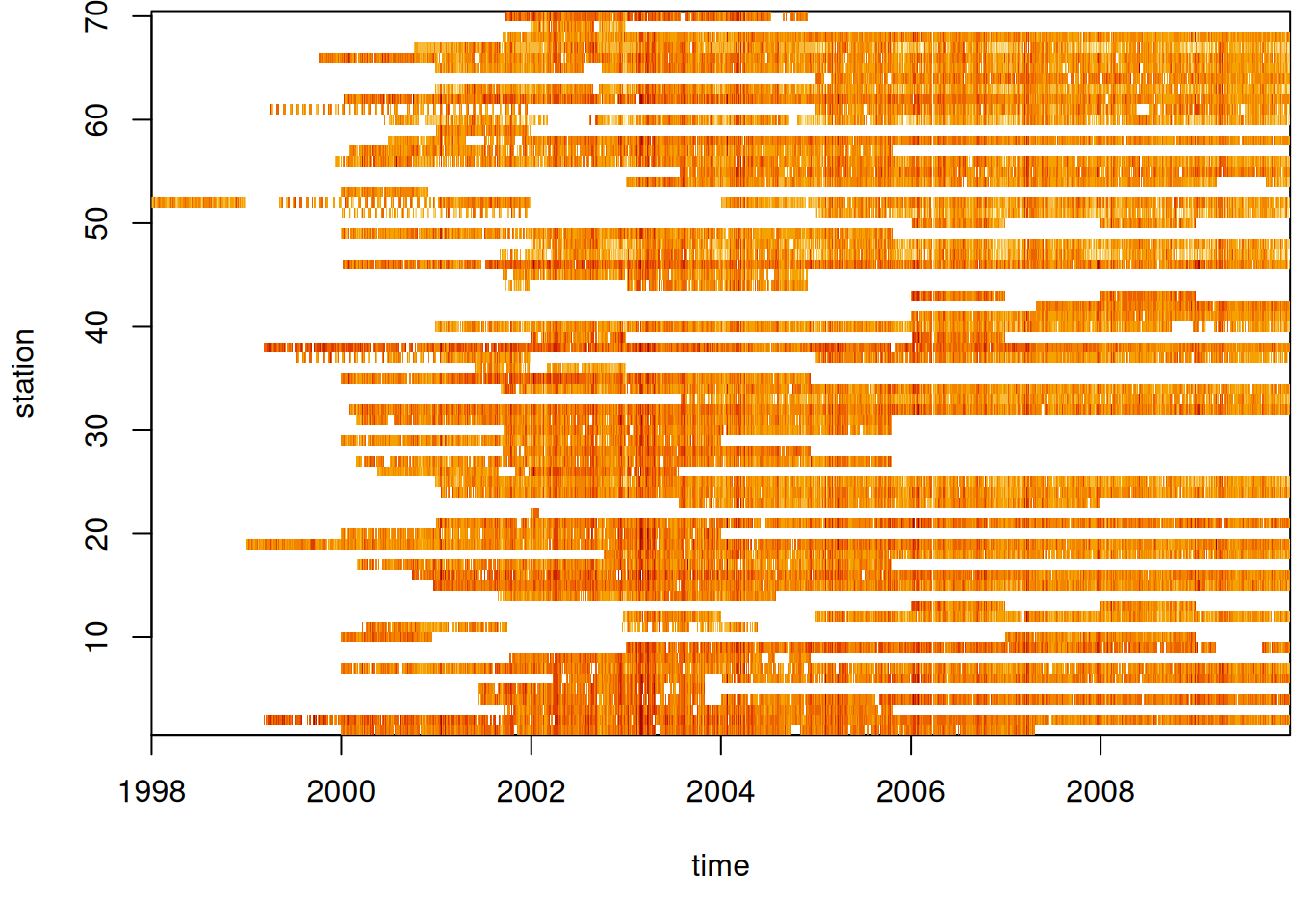Figure 7.11: Space time diagram of PM$$_{10}$$ measurements by time and station Code de_nuts1 <- read_sf("data/de_nuts1.gpkg") st_as_sf(st_apply(aq, 1, mean, na.rm = TRUE)) |> plot(reset = FALSE, pch = 16, extent = de_nuts1) st_union(de_nuts1) |> plot(add = TRUE)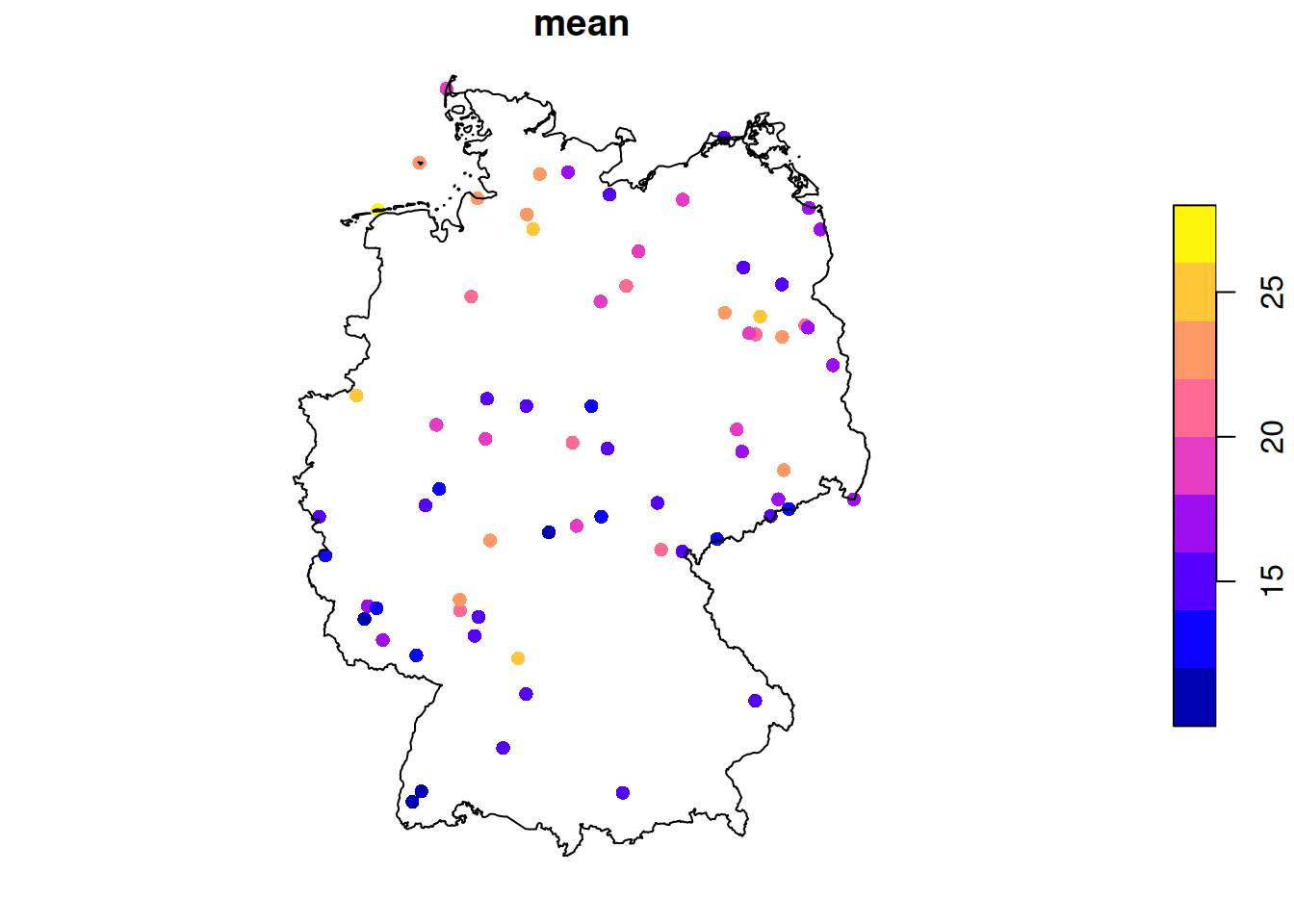Figure 7.12: Locations of PM$$_{10}$$ measurement stations, showing mean values We can aggregate these station time series to area means, mostly as a simple exercise. For this, we use the aggregate method for stars objects (a <- aggregate(aq, de_nuts1, mean, na.rm = TRUE)) # stars object with 2 dimensions and 1 attribute # attribute(s): # Min. 1st Qu. Median Mean 3rd Qu. Max. NA's # PM10 1.08 10.9 15.3 17.9 21.8 172 25679 # dimension(s): # from to offset delta refsys point # geom 1 16 NA NA WGS 84 FALSE # time 1 4383 1998-01-01 1 days Date FALSE # values # geom MULTIPOLYGON (...,...,MULTIPOLYGON (... # time NULL and we can now show the maps for six arbitrarily chosen days (Figure 7.13), using library(tidyverse) a |> filter(time >= "2008-01-01", time < "2008-01-07") |> plot(key.pos = 4)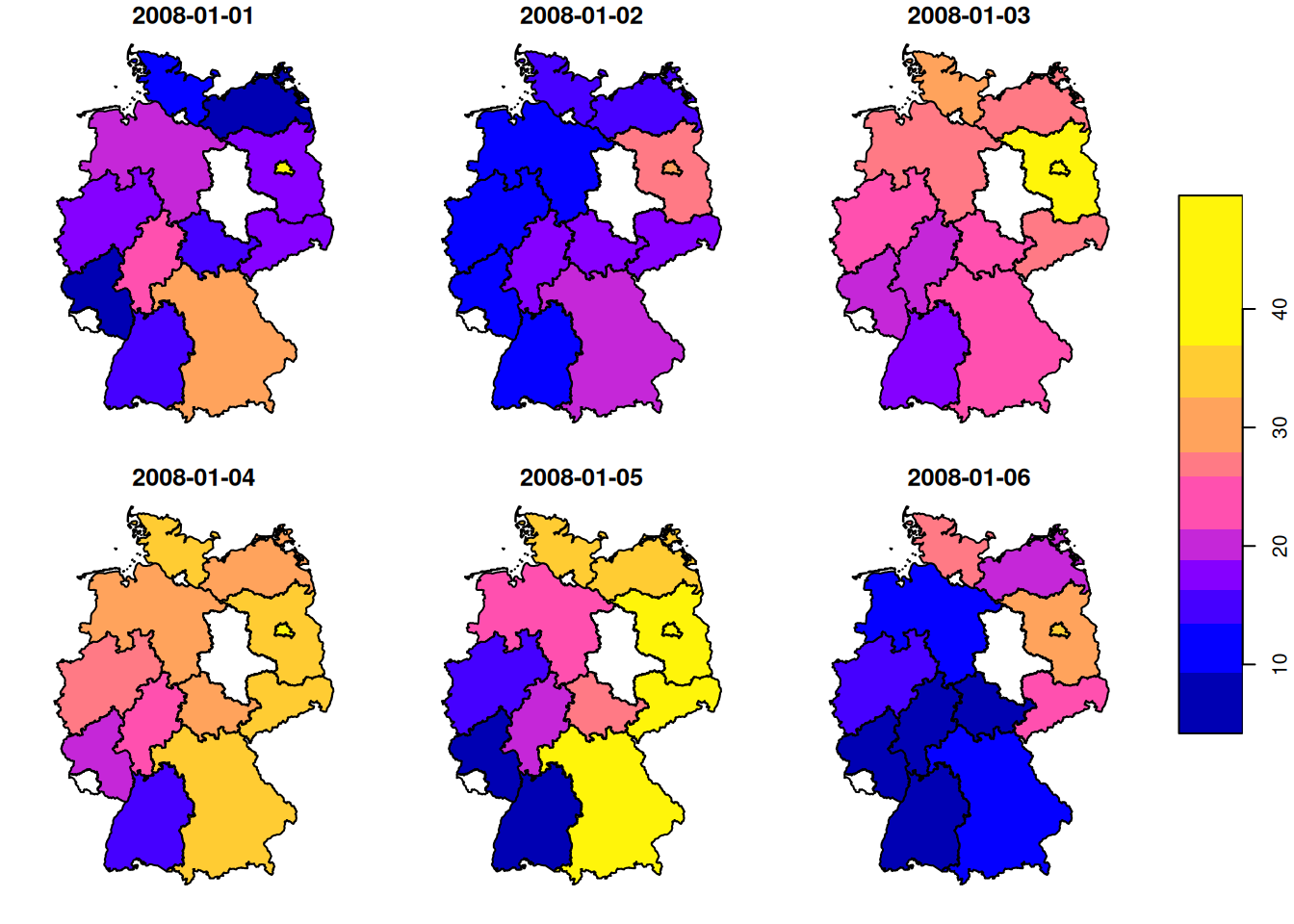Figure 7.13: Areal mean PM$$_{10}$$ values, for six days or create a time series plot of mean values for a single state (Figure 7.14) by library(xts) |> suppressPackageStartupMessages() plot(as.xts(a)[,4], main = de_nuts1NAME_1)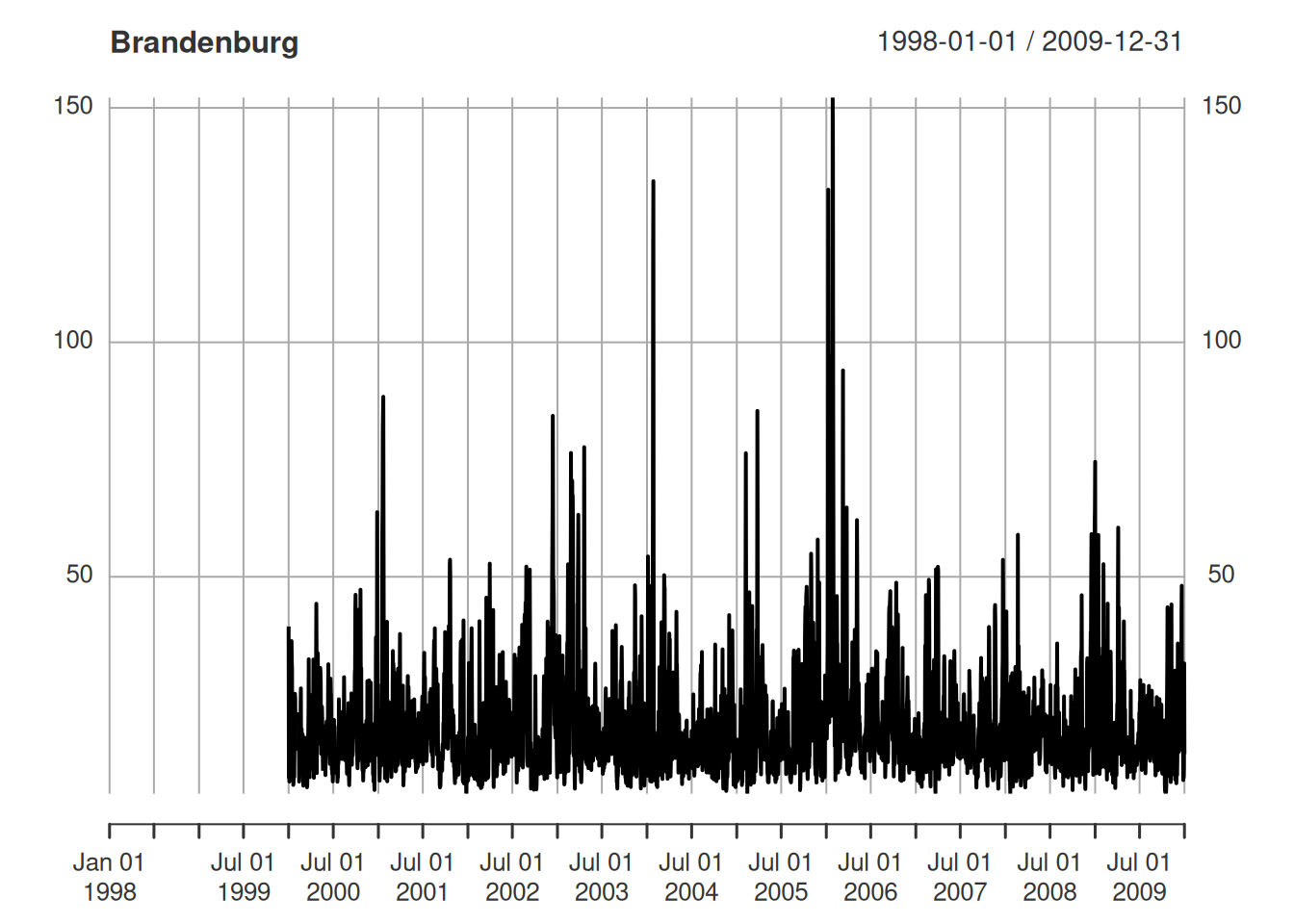Figure 7.14: Areal mean PM$$_{10}$$ time series for a single state

### Example: Bristol origin-destination data cube

The data used for this example come from Lovelace, Nowosad, and Muenchow (2019), and concern origin-destination (OD) counts: the number of persons going from zone A to zone B, by transportation mode. We have feature geometries in sf object bristol_zones for the 102 origin and destination regions, shown in Figure 7.15.

Code
library(spDataLarge)
plot(st_geometry(bristol_zones), axes = TRUE, graticule = TRUE)
plot(st_geometry(bristol_zones), col = 'red', add = TRUE)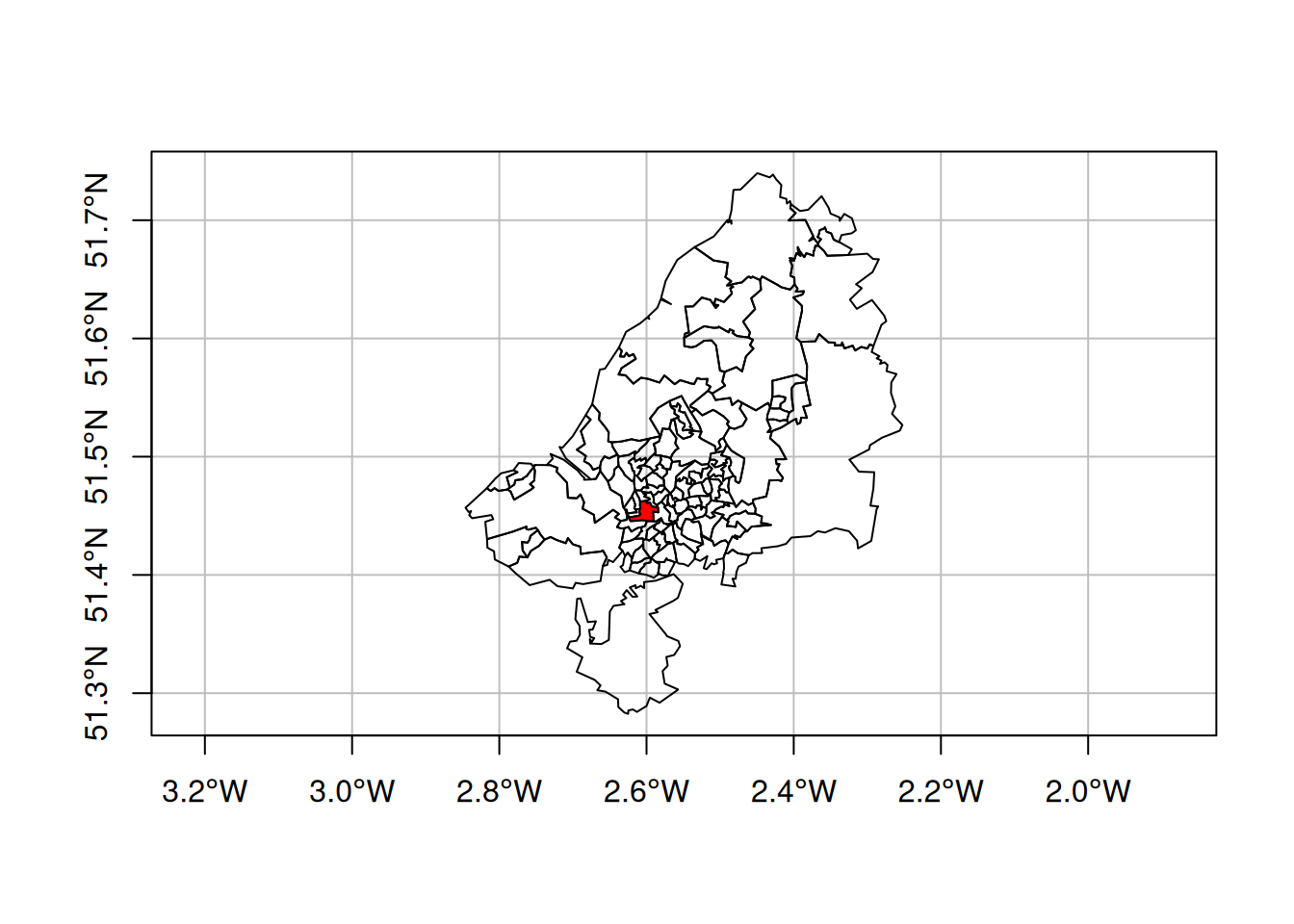Figure 7.15: Origin destination data zones for Bristol, UK, with zone 33 (E02003043) coloured red

OD counts come in a table bristol_od with non-zero OD pairs as records, and transportation mode as variables:

head(bristol_od)
# # A tibble: 6 × 7
#   o         d           all bicycle  foot car_driver train
#   <chr>     <chr>     <dbl>   <dbl> <dbl>      <dbl> <dbl>
# 1 E02002985 E02002985   209       5   127         59     0
# 2 E02002985 E02002987   121       7    35         62     0
# 3 E02002985 E02003036    32       2     1         10     1
# 4 E02002985 E02003043   141       1     2         56    17
# 5 E02002985 E02003049    56       2     4         36     0
# 6 E02002985 E02003054    42       4     0         21     0

The number of zero OD values is found by subtracting the number of non-zero records from the total number of OD combinations:

nrow(bristol_zones)^2 - nrow(bristol_od)
#  7494

We will form a three-dimensional vector data cube with origin, destination, and transportation mode as dimensions. For this, we first “tidy” the bristol_od table to have origin (o), destination (d), transportation mode (mode), and count (n) as variables, using pivot_longer:

# create O-D-mode array:
bristol_tidy <- bristol_od |>
select(-all) |>
pivot_longer(3:6, names_to = "mode", values_to = "n")
# # A tibble: 6 × 4
#   o         d         mode           n
#   <chr>     <chr>     <chr>      <dbl>
# 1 E02002985 E02002985 bicycle        5
# 2 E02002985 E02002985 foot         127
# 3 E02002985 E02002985 car_driver    59
# 4 E02002985 E02002985 train          0
# 5 E02002985 E02002987 bicycle        7
# 6 E02002985 E02002987 foot          35

Next, we form the three-dimensional array a, filled with zeroes:

od <- bristol_tidy |> pull("o") |> unique()
nod <- length(od)
mode <- bristol_tidy |> pull("mode") |> unique()
nmode = length(mode)
a = array(0L,  c(nod, nod, nmode),
dimnames = list(o = od, d = od, mode = mode))
dim(a)
#  102 102   4

We see that the dimensions are named with the zone names (o, d) and the transportation mode name (mode). Every row of bristol_tidy denotes a single array entry, and we can use this to fill the non-zero entries of a using the bristol_tidy table to provide index (o, d and mode) and value (n):

a[as.matrix(bristol_tidy[c("o", "d", "mode")])] <-
bristol_tidy$n To be sure that there is not an order mismatch between the zones in bristol_zones and the zone names in bristol_tidy, we can get the right set of zones by: order <- match(od, bristol_zones$geo_code)
zones <- st_geometry(bristol_zones)[order]

(It happens that the order is already correct, but it is good practice to not assume this.)

Next, with zones and modes we can create a stars dimensions object:

library(stars)
(d <- st_dimensions(o = zones, d = zones, mode = mode))
#      from  to refsys point                                  values
# o       1 102 WGS 84 FALSE MULTIPOLYGON (...,...,MULTIPOLYGON (...
# d       1 102 WGS 84 FALSE MULTIPOLYGON (...,...,MULTIPOLYGON (...
# mode    1   4     NA FALSE                       bicycle,...,train

and finally build a stars object from a and d:

(odm <- st_as_stars(list(N = a), dimensions = d))
# stars object with 3 dimensions and 1 attribute
# attribute(s):
#    Min. 1st Qu. Median Mean 3rd Qu. Max.
# N     0       0      0  4.8       0 1296
# dimension(s):
#      from  to refsys point                                  values
# o       1 102 WGS 84 FALSE MULTIPOLYGON (...,...,MULTIPOLYGON (...
# d       1 102 WGS 84 FALSE MULTIPOLYGON (...,...,MULTIPOLYGON (...
# mode    1   4     NA FALSE                       bicycle,...,train

We can take a single slice through this three-dimensional array, for instance for zone 33 (Figure 7.15), by odm[ , , 33], and plot it with

plot(adrop(odm[,,33]) + 1, logz = TRUE)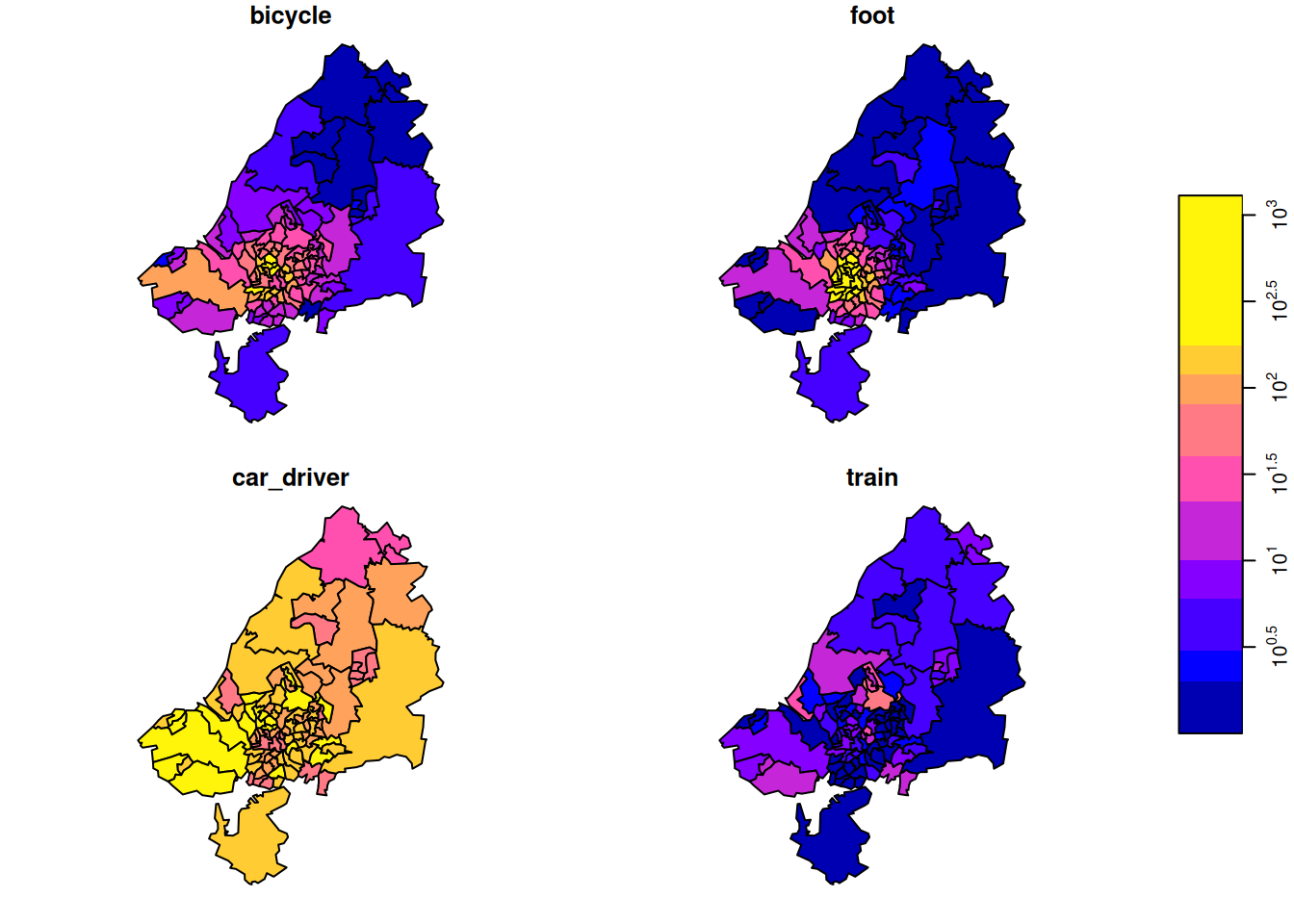Figure 7.16: OD matrix sliced for destination zone 33, by transportation mode

the result of which is shown in Figure 7.16. Subsetting this way, we take all attributes (there is only one: N) since the first argument is empty, we take all origin regions (second argument empty), we take destination zone 33 (third argument), and all transportation modes (fourth argument empty, or missing).

We plotted this particular zone because it has the largest number of travellers as its destination. We can find this out by summing all origins and travel modes by destination:

d <- st_apply(odm, 2, sum)
which.max(d[])
#  33

Other aggregations we can carry out include:

Total transportation by OD (102 x 102):

st_apply(odm, 1:2, sum)
# stars object with 2 dimensions and 1 attribute
# attribute(s):
#      Min. 1st Qu. Median Mean 3rd Qu. Max.
# sum     0       0      0 19.2      19 1434
# dimension(s):
#   from  to refsys point                                  values
# o    1 102 WGS 84 FALSE MULTIPOLYGON (...,...,MULTIPOLYGON (...
# d    1 102 WGS 84 FALSE MULTIPOLYGON (...,...,MULTIPOLYGON (...

Origin totals, by mode:

st_apply(odm, c(1,3), sum)
# stars object with 2 dimensions and 1 attribute
# attribute(s):
#      Min. 1st Qu. Median Mean 3rd Qu. Max.
# sum     1    57.5    214  490     771 2903
# dimension(s):
#      from  to refsys point                                  values
# o       1 102 WGS 84 FALSE MULTIPOLYGON (...,...,MULTIPOLYGON (...
# mode    1   4     NA FALSE                       bicycle,...,train

Destination totals, by mode:

st_apply(odm, c(2,3), sum)
# stars object with 2 dimensions and 1 attribute
# attribute(s):
#      Min. 1st Qu. Median Mean 3rd Qu.  Max.
# sum     0      13    104  490     408 12948
# dimension(s):
#      from  to refsys point                                  values
# d       1 102 WGS 84 FALSE MULTIPOLYGON (...,...,MULTIPOLYGON (...
# mode    1   4     NA FALSE                       bicycle,...,train

Origin totals, summed over modes:

o <- st_apply(odm, 1, sum)

Destination totals, summed over modes (we had this):

d <- st_apply(odm, 2, sum)

We plot o and d together after joining them by

x <- (c(o, d, along = list(od = c("origin", "destination"))))
plot(x, logz = TRUE)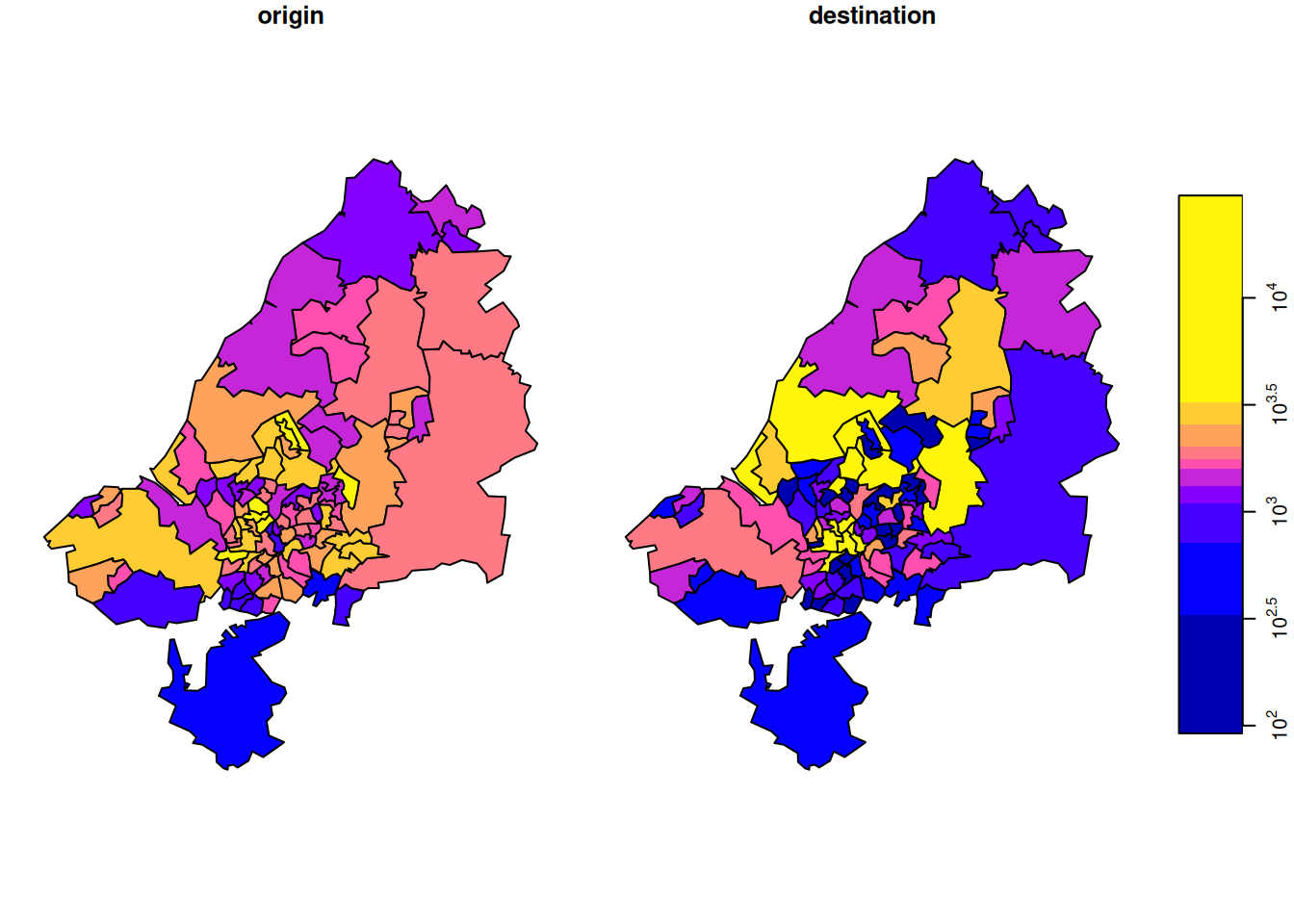Figure 7.17: Total commutes, summed by origin (left) or destination (right)

the result of which is shown in Figure 7.17.

There is something to say for the argument that such maps give the wrong message, as both amount (colour) and polygon size give an impression of amount. To take out the amount in the count, we can compute densities (count / km$$^2$$), by

library(units)
a <- set_units(st_area(st_as_sf(o)), km^2)
o$sum_km <- o$sum / a
d$sum_km <- d$sum / a
od <- c(o["sum_km"], d["sum_km"], along =
list(od = c("origin", "destination")))
plot(od, logz = TRUE)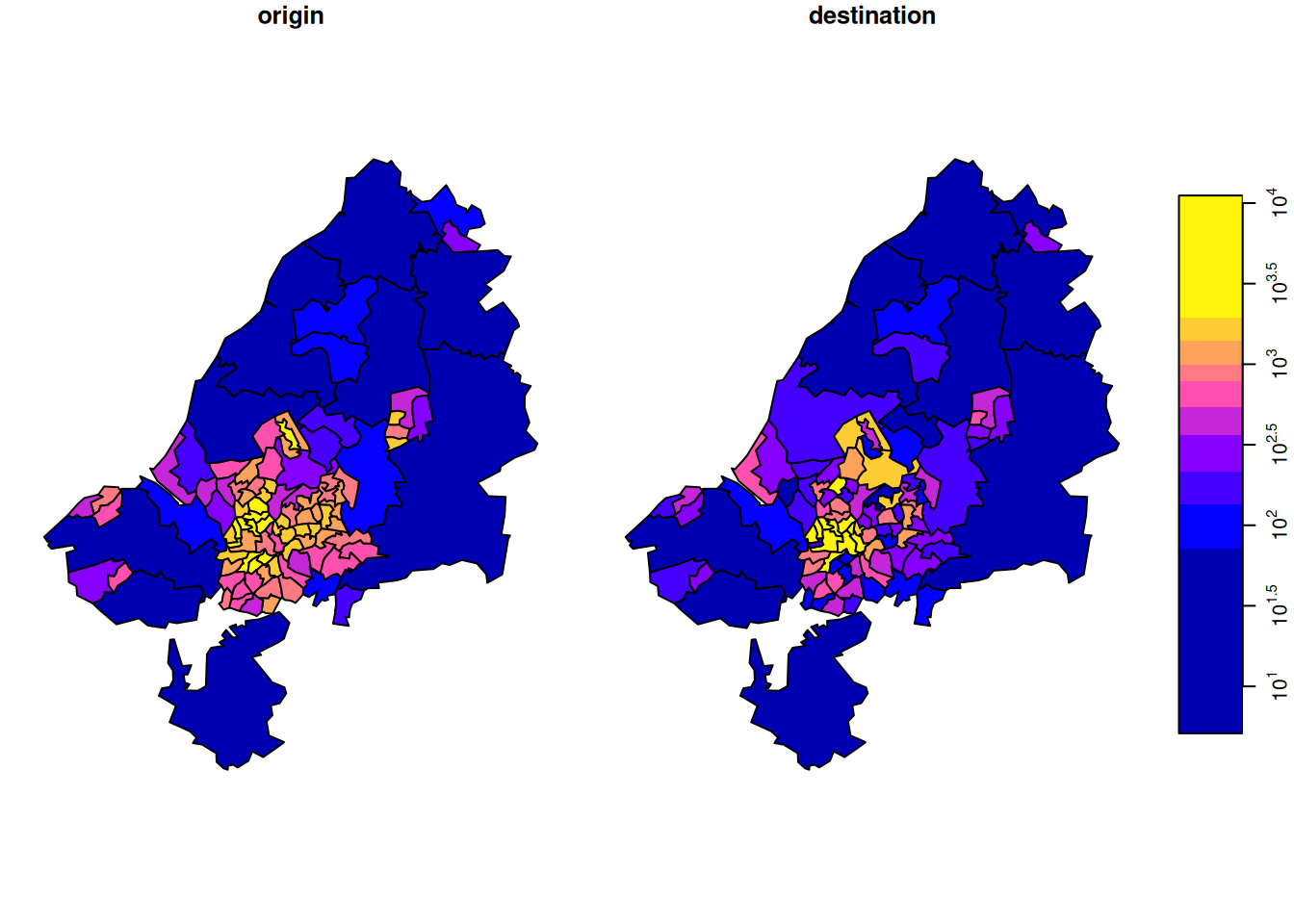Figure 7.18: Total commutes per square km, by area of origin (left) or destination (right)

shown in Figure 7.18. Another way to normalize these totals would be to divide them by population size.

### Tidy array data

The tidy data paper may suggest that such array data should be processed not as an array, but in a long (unnormalised) table form where each row holds (region, class, year, value), and it is always good to be able to do this. For primary handling and storage, however, this is often not an option, because:

• a lot of array data are collected or generated as array data, for instance by imagery or other sensory devices, or by climate models
• it is easier to derive the long table form from the array than vice versa
• the long table form requires much more memory, since the space occupied by dimension values is $$O(\Pi n_i)$$, rather than $$O(\Sigma n_i)$$, with $$n_i$$ the cardinality (size) of dimension $$i$$
• when missing-valued cells are dropped, the long table form loses the implicit indexing of the array form

To put this argument to the extreme, consider for instance that all image, video and sound data are stored in array form; few people would make a real case for storing them in a long table form instead. Nevertheless, R packages like tsibble take this approach, and have to deal with ambiguous ordering of multiple records with identical time steps for different spatial features and index them, which is solved for both automatically by using the array form – at the cost of using dense arrays, in package stars.

Package stars tries to follow the tidy manifesto to handle array sets and has particularly developed support for the case where one or more of the dimensions refer to space and/or time.

### File formats for vector data cubes

Regular table forms, including the long table form, are possible but clumsy to use: the origin-destination data example above and Chapter 13 illustrate the complexity of recreating a vector data cube from table forms. Array formats like NetCDF or Zarr are designed for storing array data. They can however be used for any data structure, and carry the risk that files once written are hard to reuse. For vector cubes that have a single geometry dimension that consists of either points, (multi)linestrings or (multi)polygons, the CF conventions describe a way to encode such geometries. stars::read_mdim and stars::write_mdim can read and write vector data cubes following these conventions.

## 7.6 Raster-to-vector, vector-to-raster

Section 1.3 already showed some examples of raster-to-vector and vector-to-raster conversions. This section will add some code details and examples.

### Vector-to-raster

st_as_stars is meant as a method to transform objects into stars objects. However, not all stars objects are raster objects, and the method for sf objects creates a vector data cube with the geometry as its spatial (vector) dimension, and attributes as attributes. When given a feature geometry (sfc) object, st_as_stars will rasterise it, as shown in Section 7.8 and in Figure 7.19.

file <- system.file("gpkg/nc.gpkg", package="sf")
st_geometry() |>
st_as_stars() |>
plot(key.pos = 4)

Here, st_as_stars can be parameterised to control cell size, number of cells, and/or extent. The cell values returned are 0 for cells with centre point outside the geometry and 1 for cell with centre point inside or on the border of the geometry. Rasterising existing features is done using st_rasterize, as also shown in Figure 1.5:

library(dplyr)
mutate(name = as.factor(NAME)) |>
select(SID74, SID79, name) |>
st_rasterize()
# stars object with 2 dimensions and 3 attributes
# attribute(s):
#      SID74           SID79            name
#  Min.   : 0      Min.   : 0      Sampson :  655
#  1st Qu.: 3      1st Qu.: 3      Columbus:  648
#  Median : 5      Median : 6      Robeson :  648
#  Mean   : 8      Mean   :10      Bladen  :  604
#  3rd Qu.:10      3rd Qu.:13      Wake    :  590
#  Max.   :44      Max.   :57      (Other) :30952
#  NA's   :30904   NA's   :30904   NA's    :30904
# dimension(s):
#   from  to offset   delta refsys point x/y
# x    1 461  -84.3  0.0192  NAD27 FALSE [x]
# y    1 141   36.6 -0.0192  NAD27 FALSE [y]

Similarly, line and point geometries can be rasterised, as shown in Figure 7.20.

read_sf(file) |>
st_cast("MULTILINESTRING") |>
select(CNTY_ID) |>
st_rasterize() |>
plot(key.pos = 4)

## 7.7 Coordinate transformations and conversions

### st_crs

Spatial objects of class sf or stars contain a coordinate reference system that can be retrieved or replaced with st_crs, or can be set or replaced in a pipe with st_set_crs. Coordinate reference systems can be set with an EPSG code, like st_crs(4326) which will be converted to st_crs('EPSG:4326'), or with a PROJ.4 string like "+proj=utm +zone=25 +south", a name like “WGS84”, or a name preceded by an authority like “OGC:CRS84”. Alternatives include a coordinate reference system definition in WKT, WKT-2 (Section 2.5) or PROJJSON. The object returned by st_crs contains two fields:

• wkt with the WKT-2 representation
• input with the user input, if any, or a human readable description of the coordinate reference system, if available

Note that PROJ.4 strings can be used to define some coordinate reference systems, but they cannot be used to represent coordinate reference systems. Conversion of a WKT-2 in a crs object to a proj4string using the $proj4string method, as in x <- st_crs("OGC:CRS84") x$proj4string
#  "+proj=longlat +datum=WGS84 +no_defs"

may succeed but is not in general lossless or invertible. Using PROJ.4 strings, for instance to define a parameterised, projected coordinate reference system is fine as long as it is associated with the WGS84 datum.

### st_transform, sf_project

Coordinate transformations or conversions (Section 2.4) for sf or stars objects are carried out with st_transform, which takes as its first argument a spatial object of class sf or stars that has a coordinate reference system set, and as a second argument with an crs object (or something that can be converted to it with st_crs). When PROJ finds more than one possibility to transform or convert from the source crs to the target crs, it chooses the one with the highest declared accuracy. More fine-grained control over the options is explained in Section 7.7.5. For stars objects with regular raster dimensions, st_transform will only transform coordinates and always result in a curvilinear grid. st_warp can be used to create a regular raster in a new coordinate reference system, using regridding (Section 7.8).

A lower-level function to transform or convert coordinates not in sf or stars objects is sf_project: it takes a matrix with coordinates and a source and target crs, and it returns transformed or converted coordinates.

### sf_proj_info

Function sf_proj_info can be used to query the available projections, ellipsoids, units and prime meridians available in the PROJ software. It takes a single parameter, type, which can have the following values:

• type = "proj" lists the short and long names of available projections; short names can be used in a “+proj=name” string
• type = "ellps" lists the available ellipses, with name, long name, and ellipsoidal parameters
• type = "units" lists the available length units, with conversion constant to meters
• type = "prime_meridians" lists the prime meridians with their position with respect to the Greenwich meridian

### Datum grids, proj.db, cdn.proj.org, local cache

Datum grids (Section 2.4) can be installed locally, or be read from the PROJ datum grid CDN at https://cdn.proj.org/. If installed locally, they are read from the PROJ search path, which is shown by

sf_proj_search_paths()
#  "/home/edzer/.local/share/proj" "/usr/share/proj"

The main PROJ database is proj.db, an sqlite3 database typically found at

### Axis order and direction

As mentioned in Section 2.5, EPSG:4326 defines the first axis to be associated with latitude and the second with longitude; this is also the case for a number of other ellipsoidal coordinate reference systems. Although this is how the authority (EPSG) prescribes this, it is not how most datasets are currently stored. As most other software, package sf by default ignores this and interprets ellipsoidal coordinate pairs as (longitude, latitude) by default. If however data needs to be read from a data source that is compliant to the authority, for instance from a WFS service, one can set

st_axis_order(TRUE)

to globally instruct sf, when calling GDAL and PROJ routines, that authority compliance (latitude, longitude order) is assumed. It is anticipated that problems may happen in case of authority compliance, for instance when with plotting data. The plot method for sf objects respects the axis order flag and will swap coordinates using the transformation pipeline "+proj=pipeline +step +proj=axisswap +order=2,1" before plotting them, but geom_sf in ggplot2 has not been modified to do this. As mentioned earlier, the axis order ambiguity of EPSG:4326 is resolved by replacing it with OGC:CRS84.

Independent from the axis order, not all coordinate reference systems have an axis direction that is positive in North and East directions. Most plotting functions in R will not work with data that have axes defined in opposite directions. Information on axes directions and units can be retrieved by

st_crs(4326)$axes # name orientation # 1 Geodetic latitude 1 # 2 Geodetic longitude 3 st_crs(4326)$ud_unit
# 1 [°]
st_crs("EPSG:2053")$axes # name orientation # 1 Westing 4 # 2 Southing 2 st_crs("EPSG:2053")$ud_unit
# 1 [m]

## 7.8 Transforming and warping rasters

When using st_transform on a raster dataset, as in

tif <- system.file("tif/L7_ETMs.tif", package = "stars")
st_transform('OGC:CRS84')
# stars object with 3 dimensions and 1 attribute
# attribute(s):
#              Min. 1st Qu. Median Mean 3rd Qu. Max.
# L7_ETMs.tif     1      54     69 68.9      86  255
# dimension(s):
#      from  to refsys point                    values x/y
# x       1 349 WGS 84 FALSE [349x352] -34.9,...,-34.8 [x]
# y       1 352 WGS 84 FALSE [349x352] -8.04,...,-7.95 [y]
# band    1   6     NA    NA                      NULL
# curvilinear grid

we see that a curvilinear is created, which means that for every grid cell the coordinates are computed in the new CRS, which no longer form a regular grid. Plotting such data is extremely slow, as small polygons are computed for every grid cell and then plotted. The advantage of this is that no information is lost: grid cell values remain identical after the projection.

When we start with a raster on a regular grid and want to obtain a regular grid in a new coordinate reference system, we need to warp the grid: we need to recreate a grid at new locations and use some rule to assign values to new grid cells. Rules can involve using the nearest value or using some form of interpolation. This operation is not lossless and not invertible.

The best approach for warping is to specify the target grid as a stars object. When only a target CRS is specified, default options for the target grid are picked that may be completely inappropriate for the problem at hand. An example workflow that uses only a target CRS is

read_stars(tif) |>
st_warp(crs = st_crs('OGC:CRS84')) |>
st_dimensions()
#      from  to offset     delta refsys x/y
# x       1 350  -34.9  0.000259 WGS 84 [x]
# y       1 352  -7.95 -0.000259 WGS 84 [y]
# band    1   6     NA        NA     NA

which creates a pretty close raster, but then the transformation is also relatively modest. For a workflow that creates a target raster first, here with exactly the same number of rows and columns as the original raster, one could use:

r <- read_stars(tif)
grd <- st_bbox(r) |>
st_as_sfc() |>
st_transform('OGC:CRS84') |>
st_bbox() |>
st_as_stars(nx = dim(r)["x"], ny = dim(r)["y"])
st_warp(r, grd)
# stars object with 3 dimensions and 1 attribute
# attribute(s):
#              Min. 1st Qu. Median Mean 3rd Qu. Max. NA's
# L7_ETMs.tif     1      54     69 68.9      86  255 6180
# dimension(s):
#      from  to offset     delta refsys x/y
# x       1 349  -34.9   0.00026 WGS 84 [x]
# y       1 352  -7.95 -0.000259 WGS 84 [y]
# band    1   6     NA        NA     NA

where we see that grid resolution in $$x$$ and $$y$$ directions slightly varies.

## 7.9 Exercises

Use R to solve the following exercises.

1. Find the names of the nc counties that intersect LINESTRING(-84 35,-78 35); use [ for this, and as an alternative use st_join for this.
2. Repeat this after setting sf_use_s2(FALSE), and compute the difference (hint: use setdiff), and colour the counties of the difference using colour ‘#88000088’.
3. Plot the two different lines in a single plot; note that R will plot a straight line always straight in the projection currently used; st_segmentize can be used to add points on a straight line, or on a great circle for ellipsoidal coordinates.
4. NDVI, normalised differenced vegetation index, is computed as (NIR-R)/(NIR+R), with NIR the near infrared and R the red band. Read the L7_ETMs.tif file into object x, and distribute the band dimensions over attributes by split(x, "band"). Then, add attribute NDVI to this object by using an expression that uses the NIR (band 4) and R (band 3) attributes directly.
5. Compute NDVI for the L7_ETMs.tif image by reducing the band dimension, using st_apply and a function ndvi = function(x) { (x-x)/(x+x) }. Plot the result, and write the result to a GeoTIFF.
6. Use st_transform to transform the stars object read from L7_ETMs.tif to OGC:CRS84. Print the object. Is this a regular grid? Plot the first band using arguments axes=TRUE and border=NA, and explain why this takes such a long time.
7. Use st_warp to warp the L7_ETMs.tif object to OGC:CRS84, and plot the resulting object with axes=TRUE. Why is the plot created much faster than after st_transform?
8. Using a vector representation of the raster L7_ETMs, plot the intersection with a circular area around POINT(293716 9113692) with radius 75 m, and compute the area-weighted mean pixel values for this circle. Compare the area-weighted values with those obtained by aggregate using the vector data, and by aggregate using the raster data, using exact=FALSE (default) and exact=TRUE`. Explain the differences.